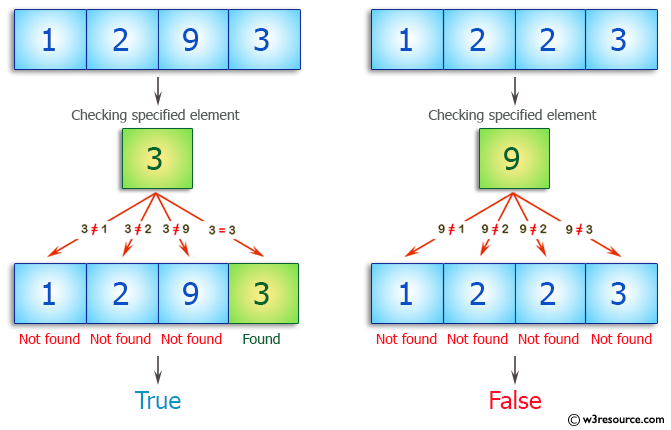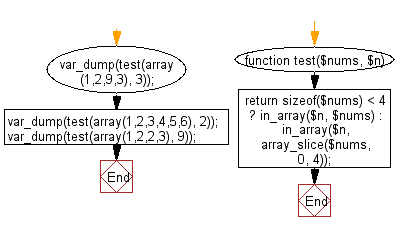﻿ PHP Exercises: Check if one of the first 4 elements in an array of integers is equal to a given element - w3resource# PHP Exercises: Check if one of the first 4 elements in an array of integers is equal to a given element

## PHP Basic Algorithm: Exercise-33 with Solution

Write a PHP program to check if one of the first 4 elements in an array of integers is equal to a given element.

Sample Solution:

PHP Code :

``````<?php
function test(\$nums, \$n)
{
return sizeof(\$nums) < 4 ? in_array(\$n, \$nums) : in_array(\$n, array_slice(\$nums, 0, 4));
}

var_dump(test(array(1,2,9,3), 3));
var_dump(test(array(1,2,3,4,5,6), 2));
var_dump(test(array(1,2,2,3), 9));
``````

Sample Output:

```bool(true)
bool(true)
bool(false)
```

Pictorial Presentation:Flowchart:PHP Code Editor:

What is the difficulty level of this exercise?

﻿

## PHP: Tips of the Day

\$_REQUEST: This SuperGlobal Variable is used to collect data submitted by a HTML Form

Example:

This code save in a php file and run in the browser.

```<!DOCTYPE html>
<html>
<body>

<form method="post" action="<?php echo \$_SERVER['PHP_SELF'];?>">
NAME: <input type="text" name="user">
<button type="submit">SUBMIT</button>
</form>
<?php
if (\$_SERVER["REQUEST_METHOD"] == "POST") {
\$name = htmlspecialchars(\$_REQUEST['user']);
if(empty(\$name)){
echo "Name is empty";
} else {
echo \$name;
}
}
?>
</body>
</html>
```

Output:

```Owen
```